Courses

# Test: Unit Digits- 3

## 10 Questions MCQ Test Additional Questions for GMAT Practice (Quant and Verbal) | Test: Unit Digits- 3

Description
This mock test of Test: Unit Digits- 3 for UPSC helps you for every UPSC entrance exam. This contains 10 Multiple Choice Questions for UPSC Test: Unit Digits- 3 (mcq) to study with solutions a complete question bank. The solved questions answers in this Test: Unit Digits- 3 quiz give you a good mix of easy questions and tough questions. UPSC students definitely take this Test: Unit Digits- 3 exercise for a better result in the exam. You can find other Test: Unit Digits- 3 extra questions, long questions & short questions for UPSC on EduRev as well by searching above.
QUESTION: 1

### Given that a, b, c, and d are different nonzero digits and that 10d + 11c < 100 – a, which of the following could not be a solution to the addition problem below?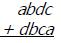Solution:

In digit problems, it is usually best to find some characteristic that must be true of the correct solution. In looking at the given addition problem, the only promising feature is that the digit b is in the hundredths place in both numbers that are being added.
What does this mean? Adding together two of the same numbers is the same as multiplying the number by 2. In other words, b + b = 2b. This implies that the hundredths place in the correct solution should be an even number (since all multiples of 2 are even).
However, this implication is ONLY true if their is no "carry over" into the hundredths column. If the addition of the units and tens digits requires us to "carry over" a 1 into the hundredths column, then this will throw off our logic. Instead of just adding b + b to form the hundredths digit of the solution, we will be adding 1 + b + b (which would sum to an odd digit in the hundredths place of the solution).
The question then becomes, will there be a "carry over" into the hundredths column? If not, then the hundredths digit of the solution MUST be even. If there is a carry over, then the hundredths digit of the solution MUST be odd.
The only way that there would be a "carry over" into the hundredths column is if the sum of the units and tens places is equal to 100 or greater.
The sum of the units place can be written as c + a.
The sum of the tens place can be written as 10d + 10c.
Thus, the sum of the units and tens places can be written as c + a + 10d + 10c which simplifies to 10d + 11c + a.
The problem states that 10d + 11c < 100 – a. This can be rewritten as 10d + 11c + a < 100. In other words, the sum of the units and ten places totals to less than 100. Therefore, there is no "carry over" into the hundredths column and so the hundredths digit of the solution MUST be even.
The problem asks us which of the answer choices could NOT be a solution to the given addition problem, so we simply need to find an answer choice that does NOT have an even number in the hundredths place.
The only answer choice that qualifies is 8581. The correct answer is C

QUESTION: 2

###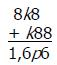If k and p represent non-zero digits within the integers above, what is p?

Solution:

Solving this problem requires a bit of logic.  A quick look at the ones column tells us that a value of 1 must be 'carried' to the tens column.  As a result, p must equal the ones digit from the sum of k + 8 + 1, or k + 9 (note that it would be incorrect to say that p = k + 9).
Now, given that k is a non-zero digit, k + 9 must be greater than or equal to 10.  Furthermore, since k is a single digit and must be less than 10, we can also conclude that k + 9 < 20.  Therefore, we know that a value of 1 will be 'carried' to the hundreds column as well.
We are now left with some basic algebra. In the hundreds column, 8 + k + 1 = 16, so k = 7.  Recall that p equals the ones digit of k + 9.  k + 9 = 7 + 9 = 16, so p = 6.

QUESTION: 3

### If x represents the sum of all the positive three-digit numbers that can be constructed using each of the distinct nonzero digits a, b, and c exactly once, what is the largest integer by which x must be divisible?

Solution:

There are 3!, or 6, different three-digit numbers that can be constructed using the digits a, b, and c: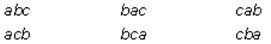The value of any one of these numbers can be represented using place values. For example, the value of abc is 100a + 10b + c.
Therefore, you can represent the sum of the 6 numbers as: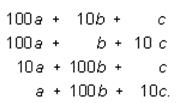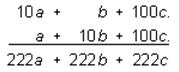=222(a+b+c)
x is equal to 222(a + b + c). Therefore, x must be divisible by 222.

QUESTION: 4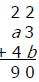If a and b represent positive single digits in the correctly worked computation above, what is the value of the two digit integer ba?

Solution:

The sum of the units digit is 2 + 3 + b = 10. We know that 2 + 3 + b can’t equal 0, because b is a positive single digit. Likewise 2 + 3 + b can’t equal 20 (or any higher value) because b would need to be 15 or greater—not a single digit.   Therefore, b must equal 5.
We know that 1 is carried from the sum of the units digits and added to the 2, a, and 4 in the tens digit of the computation, and that those digits sum to 9. Therefore 1 + 2 + a + 4 = 9, or a = 2.
Thus, the value of the two digit integer ba is 52.

QUESTION: 5

For any four digit number, abcd, *abcd*= (3a)(5b)(7c)(11d). What is the value of (n – m) if m and n are fourdigit numbers for which *m* = (3r)(5s)(7t)(11u) and *n* = (25)(*m*)?

Solution:

According to the question, the “star function” is only applicable to four digit numbers. The function takes the thousands, hundreds, tens and units digits of a four-digit number and applies them as exponents for the bases 3, 5, 7 and 11, respectively, yielding a value which is the product of these exponential expressions.
Let’s illustrate with a few examples:
*2234* = (32)(52)(73)(114)
*3487* = (33)(54)(78)(117)

According to the question, the four-digit number m must have the digits of rstu, since *m* = (3r)(5s)(7t)(11u).

If *n* = (25)(*m*)
*n* = (52)(3r)(5s)(7t)(11u)
*n* = (3r)(5s+2)(7t)(11u)

n is also a four digit number, so we can use the *n* value to identify the digits of n:
thousands =  r, hundreds = s + 2, tens = t, units = u.
All of the digits of n and m are identical except for the hundreds digits. The hundreds digits of n is two more than that of m, so n – m = 200.

QUESTION: 6

What is the unit digit in the product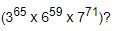Solution:

Unit digit in 34 = 1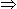Unit digit in (34)16 = 1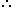Unit digit in 365 = Unit digit in [ (34)16 x 3 ] = (1 x 3) = 3
Unit digit in 659 = 6
Unit digit in 74Unit digit in (74)17 is 1.
Unit digit in 771 = Unit digit in [(74)17 x 73] = (1 x 3) = 3Required digit = Unit digit in (3 x 6 x 3) = 4.

QUESTION: 7

3251 + 587 + 369 - ? = 3007

Solution: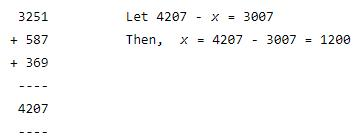QUESTION: 8

7589 - ? = 3434

Solution:

Let 7589 -x = 3434

Then, x = 7589 - 3434 = 4155

QUESTION: 9

217 x 217 + 183 x 183 = ?

Solution:

(217)2 + (183)2= (200 + 17)2 + (200 - 17)2
= 2 x [(200)2 + (17)2]
[Ref: (a + b)2 + (a - b)2 = 2(a2 + b2)]
= 2[40000 + 289]
= 2 x 40289
= 80578.

QUESTION: 10

The unit digit in the product (784 x 618 x 917 x 463) is:

Solution:

Unit digit in the given product = Unit digit in (4 x 8 x 7 x 3) = (672) = 2